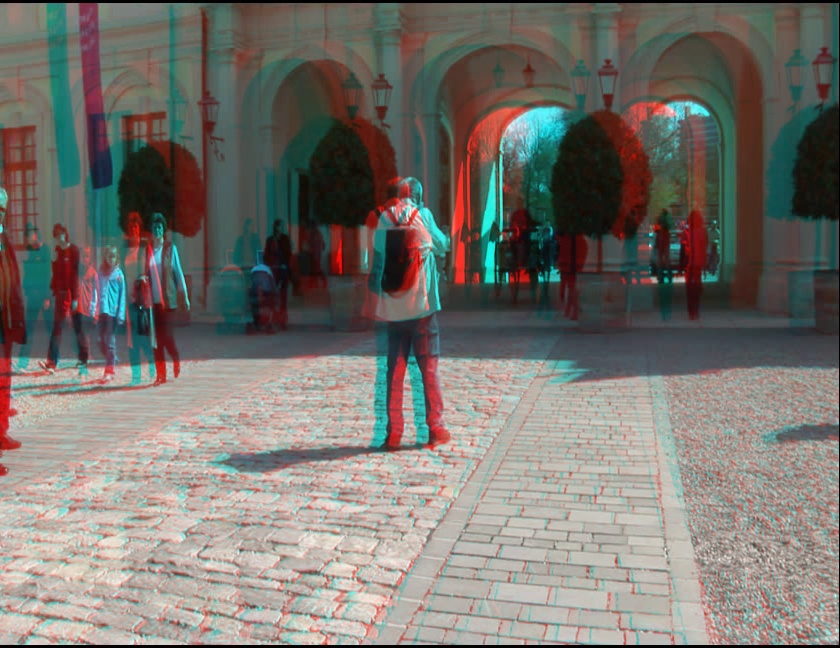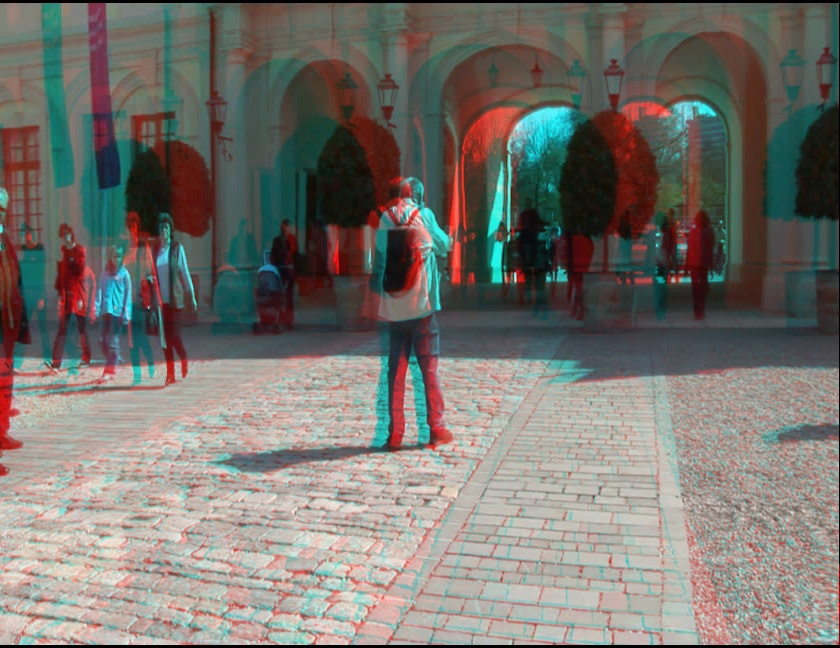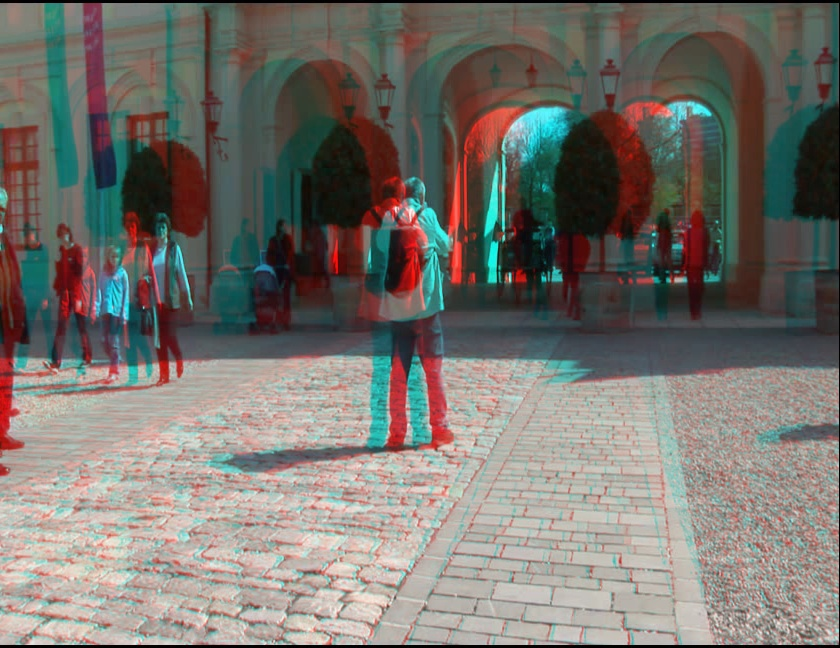Homepage

Depth Mapping for Stereoscopic Videos

Tao Yan, Rynson W.H. Lau, Yun Xu, and Liusheng Huang

Abstract
Stereoscopic videos have become very popular in recent years. Most of these videos are developed primarily for viewing on large screens located at some distance away from the viewer. If we watch these videos on a small screen located near to us, the depth range of the videos will be seriously reduced, which can significantly degrade the 3D effects of these videos. To address this problem, we propose a linear depth mapping method to adjust the depth range of a stereoscopic video according to the viewing configuration, including pixel density and distance to the screen. Our method tries to minimize the distortion of stereoscopic image contents after depth mapping, by preserving the relationship of neighboring features and preventing line and plane bending. It also considers the depth and motion coherences. While depth coherence ensures smooth changes of the depth field across frames, motion coherence ensures smooth content changes across frames. Our experimental results show that the proposed method can improve the stereoscopic effects while maintaining the quality of the output videos. [download paper](a) (b) (c) (d)

Fig 1. (a) shows the origianl stereo image, and (b)(c)(d) show the results after remapping to different depth ranges using our method. (b) and (c) remap the depth range to cover regions in front of and behind the screen, with (c) having a larger depth range than (b). (d) simply enlarges the depth range. We can see that image features, such as walls on the building, the stone road and the foor, are all well-preserved. The frame size is 648*840.

This page contains the videos that we used in our user study to evaluate the performance of our proposed depth mapping method.

In all our experiments, the viewing configuration is set as follows: the distance between the viewer and the screen is 50cm and the pixel density is 3.41pixel/mm. Image/video resolutions are specified as Height * Width.

Videos used in Experiment 1:

In this experiment, we compare the original videos with the output videos from our method. Each video combines the original frames in the left and the output frames in the right to form combined frames.

video number original video and our result retinal disparity limits
1
η1=0, η2=3
2
η1=0, η2=2
3
η1=0, η2=3
4
η1=-2, η2=4
5
η1=-1, η2=3
6
η1=0, η2=2
7
η1=0, η2=2
8
η1=0, η2=2
9
η1=0, η2=2
10
η1=0, η2=3
11
η1=0, η2=3
12
η1=-1, η2=3
13
η1=-1, η2=3
14
η1=0, η2=2
η1=0, η2=2
η1=-2, η2=2
η1=0, η2=1

Videos used in Experiment 2:

In this experiment, we compare the output videos from our method and the output videos from [Lang10]. For the output videos from our method and from [Lang10], we double the disparity ranges of original video sequence to obtain the output videos.

video number original video our result [Lang10]'s result
15
16
17